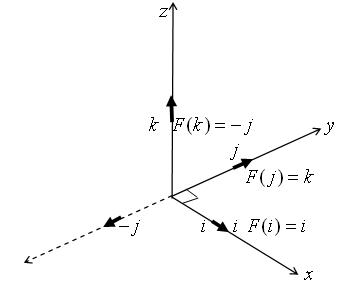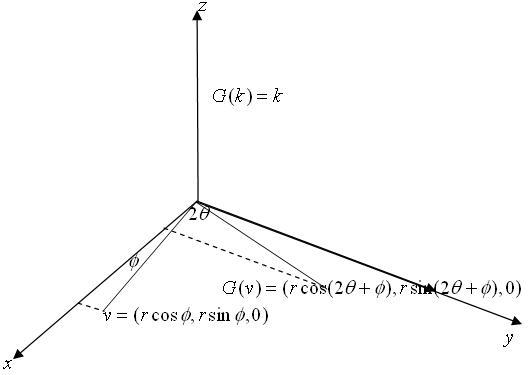#### You may also likeA quadrilateral changes shape with the edge lengths constant. Show the scalar product of the diagonals is constant. If the diagonals are perpendicular in one position are they always perpendicular?### A Knight's Journey

This article looks at knight's moves on a chess board and introduces you to the idea of vectors and vector addition.### Napoleon's Theorem

Triangle ABC has equilateral triangles drawn on its edges. Points P, Q and R are the centres of the equilateral triangles. What can you prove about the triangle PQR?

# Quaternions and Rotations

##### Age 16 to 18Challenge LevelIn this question we see how quaternions are used to give rotations of ${\bf R^3}$.(1) Consider the quaternion $$q = {1\over \sqrt 2} + {1\over \sqrt 2}{\bf i} + 0{\bf j} + 0 {\bf k}.$$ (a) Show that the multiplicative inverse of $q$ is given by $$q^{-1} = {1\over \sqrt 2} - {1\over \sqrt 2}{\bf i}$$ (b) Show that for all scalar multiples $x = t{\bf i}$ of the vector ${\bf i}$, $q x = x q$ and hence $q x q^{-1} = x$. This proves that the map $F(x) = q x q^{-1}$ fixes every point on the x axis. (c) What happens to points on the y axis under the mapping $F$? To answer this work out $F({\bf j})$. Also compute $F({\bf k})$ and show that ${\bf k} \to {\bf -j}.$
 (2) Consider the quaternion $q = \cos \theta + \sin \theta {\bf k}$ (a) Show that $\cos \theta - \sin \theta {\bf k}$ is the multiplicative inverse of $q$. (b) Show that $q{\bf k}q^{-1}={\bf k}$. (c) Show that $$q v q^{-1}= r(\cos (2\theta + \phi) {\bf i} + \sin (2\theta + \phi){\bf j})$$ where $v = (r\cos \phi {\bf i} + \sin \phi {\bf j}+0{\bf k})$ and hence that the map $G(v)= q v q^{-1}$ is a rotation about the z axis by an angle $2\theta$.To read about number systems, where quaternions fit in, why there are no three dimensional numbers and numbers in higher dimensions, see the NRICH article What Are Numbers?
If you want to know how quaternions are used in computer graphics and animation in film making read the Plus Article Maths goes to the movies .
The NRICH article The use of maths in computer games tells you a lot more about the subject. Though this article uses complex numbers and vectors and not quaternions,the mathematics is the same with quaternions which just give a shorter and neater way of writing down and working with the functions that give reflections and rotations in 3-space .